# Angles In A Pentagon

Here we will learn about angles in a pentagon, including finding the sum of the interior angles and solving problems involving interior angles and exterior angles.

There are also angles in a pentagon worksheets based on Edexcel, AQA and OCR exam questions, along with further guidance on where to go next if you’re still stuck.

## What are angles in a pentagon?

Angles in a pentagon are the angles in a fivesided polygon (2D shape).

• Regular pentagons have five equal (congruent) sides, five vertices and five equal interior angles. We can refer to this as a convex pentagon because all of the angles are less than 180^{\circ}.

• An irregular pentagon has 5 sides that are not all equal, and 5 interior angles that are not all equal.

Sum of interior angles of a pentagon is \bf{540^{\circ}}.

Sum of exterior angles of a pentagon is \bf{360^{\circ}}.

### What are angles in a pentagon?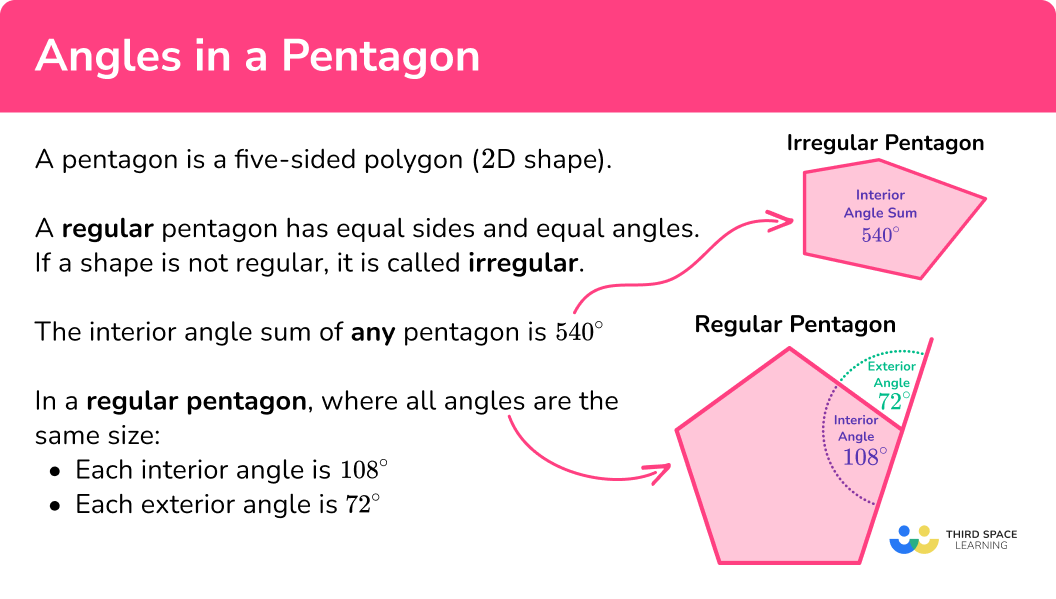### Interior and exterior angles of a pentagon

We need to be able to solve problems involving angles in a pentagon.

To do this we need to work with the interior and exterior angles of a pentagon.

A pair of interior and exterior angles of all polygons add to 180^{\circ} because they form a straight line. They are supplementary angles.

• Interior angles of a pentagon are the angles inside the 2D shape, formed when two sides of the shape meet.

We can find the sum of the interior angles of a pentagon, hexagon or any polygon using the formula,

\text{Sum of interior angles}=(n-2) \times 180, where n is the number of sides.

A pentagon has 5 sides, so n=5 .

\begin{aligned} \text{Sum of interior angles of a pentagon} & =(5-2) \times 180 \\\\ & =3 \times 180 \\\\ & =540^{\circ} \end{aligned}

To find one interior angle of a regular pentagon we can divide the sum of the interior angles by the number of sides, in this case, 5.

Step-by-step guide: Interior angles of a polygon

• Exterior angles of a pentagon are the angles between the pentagon and the extended line from the next side.

The sum of the exterior angles of any polygon is always 360^{\circ}.

To find each of the exterior angles of a regular pentagon we can use the formula,

\text{Exterior angle of a regular polygon}=\frac{360}{n}, where n is the number of sides.

Alternatively, if we know the size of the exterior angle of a regular polygon, we can use a rearrangement of the above formula to identify the number of sides the regular polygon has.

\text{number of sides}=\frac{360}{\text { exterior angle }}

Step-by-step guide: Exterior angles of a polygon

## How to solve problems involving angles in a pentagon

In order to solve problems involving angles in a pentagon:

1. Identify if the pentagon is regular or irregular.
2. Identify what the question is asking for.
3. Solve the problem using the information you have gathered.

### How to solve problems involving angles in a pentagon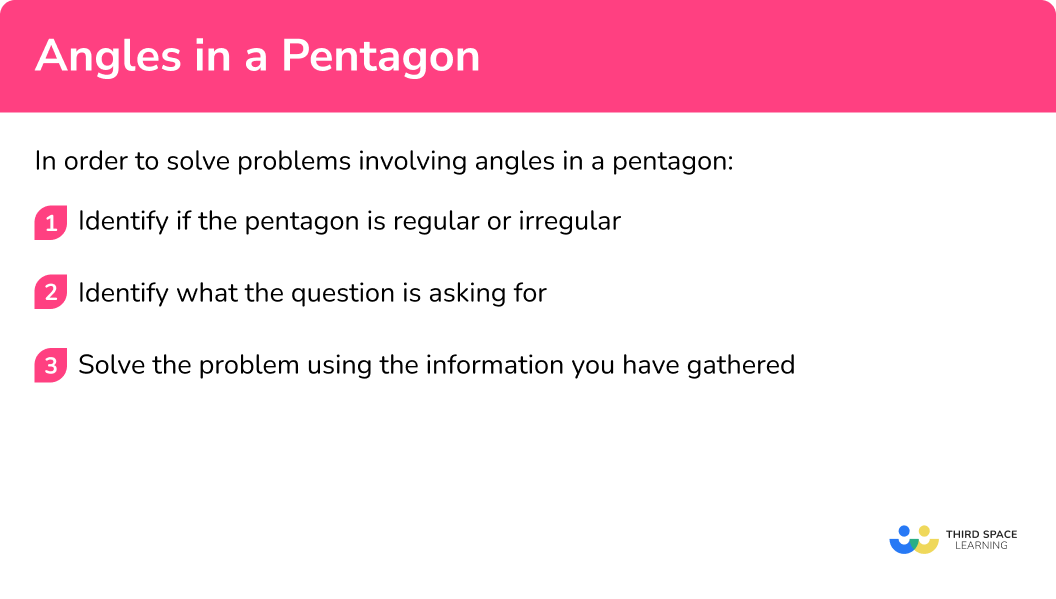### Related lessons on angles in polygons

Angles in a pentagon is part of our series of lessons to support revision on angles in polygons. You may find it helpful to start with the main angles in polygons lesson for a summary of what to expect, or use the step by step guides below for further detail on individual topics. Other lessons in this series include:

## Angles in a pentagon examples

### Example 1: finding the exterior angle of a regular pentagon

Below is a regular pentagon. Find the angle marked x.

1. Identify if the pentagon is regular or irregular.

The question tells us that this is a regular pentagon. This means the sides of the pentagon are equal and the interior angles are equal.

2Identify what the question is asking for.

The question wants us to find the angle x, which is one of the five exterior angles of this pentagon.

3Solve the problem using the information you have gathered.

To find the size of the exterior angles of a regular pentagon we can use the formula,

\text{Exterior angle of a regular polygon}=\frac{360}{n}, where n is the number of sides.

So, the missing angle can be calculated as

x=\frac{360}{5}=72^{\circ} .

So, the exterior angle of a pentagon equals 72^{\circ}.

### Example 2: finding the sum of the interior angles of a pentagon

What is the sum of the interior angles of a pentagon?

Identify if the pentagon is regular or irregular.

Identify what the question is asking for.

Solve the problem using the information you have gathered.

### Example 3: finding an interior angle of a regular pentagon

Below is a regular pentagon. Find the angle marked x.

Identify if the pentagon is regular or irregular.

Identify what the question is asking for.

Solve the problem using the information you have gathered.

### Example 4: finding an interior angle of an irregular pentagon

Below is a polygon. Find the size of the missing angle x.

Identify if the pentagon is regular or irregular.

Identify what the question is asking for.

Solve the problem using the information you have gathered.

### Example 5: finding an exterior angle of an irregular pentagon

Below is an irregular polygon. Find the size of the missing angle x.

Identify if the pentagon is regular or irregular.

Identify what the question is asking for.

Solve the problem using the information you have gathered.

### Example 6: solving angle problems involving pentagons

The diagram shows a shape formed by two regular polygons.

Find the size of the angle labelled x.

Identify if the pentagon is regular or irregular.

Identify what the question is asking for.

Solve the problem using the information you have gathered.

### Common misconceptions

• Regular and irregular polygons

It is important to correctly identify whether a polygon is regular or irregular, using the information given in the question or on the diagram. Do not assume one or the other.

• Using the formulae incorrectly

Whether a polygon is regular or irregular will determine how the formulae for working with interior and exterior angles can be used. For example, the formula

\text{Exterior angle of a regular polygon}=\frac{360}{n}, where n is the number of sides.

Can only be used to find the size of exterior angles of regular polygons, where all exterior angles are equal.

### Practice angles in a pentagon questions

1. Calculate the exterior angle of a regular pentagon.

72^{\circ}108^{\circ}540^{\circ}180^{\circ}We can find the size of an exterior angle of any regular polygon using the formula,

\text{Exterior angle of a regular polygon}=\frac{360}{n}, where n is the number of sides.

So, the size of exterior angles of a regular pentagon can be calculated as \frac{360}{5}=72^{\circ}.

2. Calculate the sum of the interior angles of a 5 sided polygon.

72^{\circ}108^{\circ}540^{\circ}900^{\circ}We can find the sum of the interior angles of any polygon using the formula

\text{Sum of interior angles}=(n-2) \times 180, where n  is the number of sides.

So, the sum of the interior angles of a pentagon can be calculated as

\begin{aligned} & =(n-2) \times 180 \\\\ & =(5-2) \times 180 \\\\ & =3 \times 180 \\\\ & =540^{\circ} \end{aligned}

3. The diagram shows a pentagon. Calculate the missing angle x.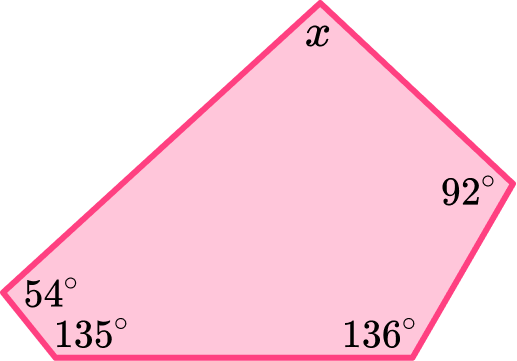108^{\circ}72^{\circ}483^{\circ}123^{\circ}To find the value of one interior angle of an irregular polygon, we first need to calculate the sum of the interior angles of the polygon.

We can find the sum of the interior angles of any polygon using the formula,

\text{Sum of interior angles}=(n-2) \times 180, where n is the number of sides.

So, the sum of the interior angles of a pentagon can be calculated as,

\begin{aligned} & =(n-2) \times 180 \\\\ & =(5-2) \times 180 \\\\ & =3 \times 180 \\\\ & =540^{\circ} \end{aligned}

Now that we know the angles inside the pentagon sum to 540^{\circ}, we can subtract the interior angles we know, to find the missing value.

x=540-(54+92+135+136)=123^{\circ}

4. The diagram shows a pentagon. Calculate the missing angle x.540^{\circ}108^{\circ}72^{\circ}116^{\circ}The interior and exterior angles of any polygon sum to 180, so,

x=180-64=116^{\circ}.

5. The diagram shows a pentagon. Calculate the missing angle x.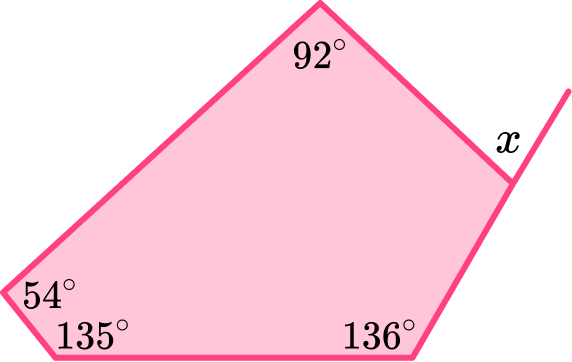108^{\circ}72^{\circ}57^{\circ}540^{\circ}To find the size of the exterior angle, x, we need to find the missing interior angle that corresponds to it. To find the size of an interior angle of any pentagon we need to first calculate the sum of the interior angles.

To calculate the sum of the interior angles of any polygon we can use the formula

\text{Sum of interior angles}=(n-2) \times 180, where n is the number of sides.

So, the interior angle of a pentagon can be calculate as,

\begin{aligned} & =(n-2) \times 180 \\\\ & =(5-2) \times 180 \\\\ & =3 \times 180 \\\\ & =540^{\circ} \end{aligned}

Now that we know the angles inside a pentagon sum to 540^{\circ}, we can subtract the interior angles we know, to find the missing value.

y=540-(92+54+135+136)=123^{\circ}

Now that we know the value of the interior angle corresponding to x, we can use the fact that the interior and exterior angles of all polygons add to 180^{\circ} because they form a straight line, to calculate the exterior angle.

So,  x=180-123=57^{\circ}.

6. The diagram below is formed by three regular polygons. Find the size of the missing value x.36^{\circ}108^{\circ}72^{\circ}144^{\circ}To find the size of the angle, x, we need to find the missing interior angles that meet it at the point.

To find the size of an interior angle of any pentagon we need to first calculate the sum of the interior angles.

To calculate the sum of the interior angles of any polygon we can use the formula,

\text{Sum of interior angles}=(n-2) \times 180, where n is the number of sides.

So, the interior angle of a pentagon can be calculate as,

\begin{aligned} & =(n-2) \times 180 \\\\ & =(5-2) \times 180 \\\\ & =3 \times 180 \\\\ & =540^{\circ} \end{aligned}

Now that we know the angles inside a pentagon sum to 540^{\circ}, we can divide this by the number of sides, in this case 5, to find the size of one of the interior angles.

So, the interior angles are

\frac{540}{5}=108^{\circ}.

Now that we know that the three interior angles are 180^{\circ} each, we can use the angle fact ‘angles around a point sum to 360^{\circ} ’ to find the angle x.

So,  x=360-(108+108+108)=36^{\circ}.

### Angles in a pentagon GCSE questions

1. Here is a regular polygon with 5 sides. Calculate the size of the interior angles of this shape.(2 marks)

(5-2)\times 180 \div 5

(1)

108^{\circ}

(1)

2. The diagram shows a pentagon. Find the missing angle x.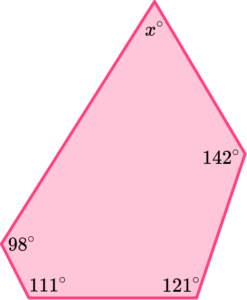(3 marks)

(5-2)\times 180=540

(1)

540-(111+98+142+121)

(1)

68^{\circ}

(1)

3. The exterior angle of a regular polygon is 72^{\circ}. Find the number of sides the polygon has, hence, name the shape.

(2 marks)

\frac{360}{72}=5

(1)

Pentagon

(1)

## Learning checklist

You have now learned how to:

• Find the exterior angle of a pentagon
• Find the the sum of the interior angles of a pentagon
• Find the value of an interior angle of a pentagon
• Solve problems involving angles in pentagons

## Still stuck?

Prepare your KS4 students for maths GCSEs success with Third Space Learning. Weekly online one to one GCSE maths revision lessons delivered by expert maths tutors.

Find out more about our GCSE maths tuition programme.